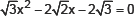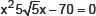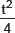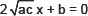# Arithmetic Progression-Important Questions

Class X Math
1.    Had Ravita scored 10 more marks in her Mathematics test out of 30 marks, 9 times these marks would have been the square of her actual marks. How many marks did she get in the test?
2.    A motor boat whose speed is 18 km/h in still water takes 1 hour more to go 24 km upstream than to return downstream to the same spot. Find the speed of the stream.
3.    In a class test, the sum of marks obtained by P in Mathematics and Science is 28. Had he got 3 more marks in Mathematics and 4 marks less in Science, the product of marks obtained in the two subjects would have been 180? Find the marks obtained in two subjects separately.
4.    Solve for x:5.    Solve for x:6.    At �t� minutes past 2 pm, the time needed by the minute hand of a clock to show 3 pm was found to be 3 minutes less thanminutes. Find ‘t’.
7.    A train, travelling at a uniform speed for 360 km, would have taken 48 minutes less to travel the same distance if its speed were 5 km/hr more. Find the original speed of the train.
8.    If the roots of the equation (b – c)x2 + (c – a)x + (a – b) = 0 are equal, then prove that 2b = a + c.
9.    If the roots of the equations
ax2 + 2bx + c = 0 and bx2are simultanously real then prove that b2 = 4ac.
10.    If the roots ff the equation
(c2 – ab)x2 – 2(a2 – bc)x + b2 – ac = 0 are equal, then prove that
either a = 0 or a3 + b3 + c3 = 3abc